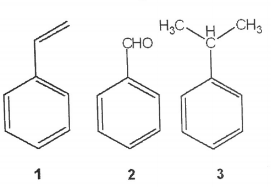# Problem: What is the correct assignment of the names of the following substituted benzenes? a. 1= anisole; 2= benzaldehyde; 3= toluene b. 1= styrene; 2= xylene; 3= toluene c. 1= aniline; 2= phenol; 3= cumene d. 1= styrene; 2= benzaldehyde; 3= cumene

###### FREE Expert Solution
93% (342 ratings)
###### Problem Details

What is the correct assignment of the names of the following substituted benzenes?

a. 1= anisole; 2= benzaldehyde; 3= toluene
b. 1= styrene; 2= xylene; 3= toluene
c. 1= aniline; 2= phenol; 3= cumene
d. 1= styrene; 2= benzaldehyde; 3= cumeneWhat scientific concept do you need to know in order to solve this problem?

Our tutors have indicated that to solve this problem you will need to apply the Naming Benzene Rings concept. You can view video lessons to learn Naming Benzene Rings. Or if you need more Naming Benzene Rings practice, you can also practice Naming Benzene Rings practice problems.

What is the difficulty of this problem?

Our tutors rated the difficulty ofWhat is the correct assignment of the names of the following...as low difficulty.

How long does this problem take to solve?

Our expert Organic tutor, Chris took 3 minutes and 40 seconds to solve this problem. You can follow their steps in the video explanation above.

What professor is this problem relevant for?

Based on our data, we think this problem is relevant for Professor Wang's class at UAB.